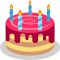### K - Blowing Candles

##### Languages: MS C# .NET 4.7.2053,GNU G++11 5.1.0 ...As Jacques-Édouard really likes birthday cakes, he celebrates his birthday every hour, instead of every year. His friends ordered him a round cake from a famous pastry shop, and placed candles on its top surface. The number of candles equals the age of Jacques-Édouard in hours. As a result, there is a huge amount of candles burning on the top of the cake. Jacques-Édouard wants to blow all the candles out in one single breath.

You can think of the flames of the candles as being points in the same plane, all within a disk of radius $R$ (in nanometers) centered at the origin. On that same plane, the air blown by Jacques-Édouard follows a trajectory that can be described by a straight strip of width $W$, which comprises the area between two parallel lines at distance $W$, the lines themselves being included in that area.  What is the minimum width $W$ such that Jacques-Édouard can blow all the candles out if he chooses the best orientation to blow?

#### Input

The first line consists of the integers $N$ and $R$, separated with a space, where $N$ is Jacques-Édouard's age in hours. Then $N$ lines follow, each of them consisting of the two integer coordinates $x_i$ and $y_i$ of the $i$th candle in nanometers, separated with a space.

Limits

• $3 \leq N \leq 2\cdot 10^{5}$;
• $10 \leq R \leq 2\cdot 10^{8}$;
• for $1\leq i\leq N$, $x^2_i + y^2_i \leq R^{2}$;
• all points have distinct coordinates.

#### Output

Print the value $W$ as a floating point number. An additive or multiplicative error of $10^{-5}$ is tolerated: if $y$ is the answer, any number either within $[y-10^{-5}; y+10^{-5}]$ or within $[(1-10^{-5})y; (1+10^{-5})y]$ is accepted.

#### Sample test(s)

Input
3 10 0 0 10 0 0 10
Output
7.0710678118654755